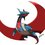# An application of newtons sum

For a given polynomial of degree $n$ with roots $r_1, r_2, \ldots r_n$, let $s_k$ be the kth symmetric sum and $p_k = \sum_{i=1}^n r_i ^k$ is the kth power sum

Claim: If $s_1=s_2=s_3=...=s_k=0$,then $p_1=p_2=p_3=.,..=p_k=0$. where

Proof: By induction on $k$. Base case: Since $s_1 = p_1$, hence if $s_1 = 0$ then $p_1 = 0$.

Induction step: Suppose that for some $j$, we know that $p_1, p_2, \ldots p_j = 0$. Then, by newtons sum, we have

$p_k=s_1p_{k-1}-s_2p_{k-2}+....+(-1)^{k-1}s_{k-2}p_2+(-1)^{k}s_{k-1}p_1+(-1)^{k+1}ks_k = 0 + 0 + \ldots + 0.$Note by Aareyan Manzoor
5 years, 9 months ago

This discussion board is a place to discuss our Daily Challenges and the math and science related to those challenges. Explanations are more than just a solution — they should explain the steps and thinking strategies that you used to obtain the solution. Comments should further the discussion of math and science.

When posting on Brilliant:

• Use the emojis to react to an explanation, whether you're congratulating a job well done , or just really confused .
• Ask specific questions about the challenge or the steps in somebody's explanation. Well-posed questions can add a lot to the discussion, but posting "I don't understand!" doesn't help anyone.
• Try to contribute something new to the discussion, whether it is an extension, generalization or other idea related to the challenge.

MarkdownAppears as
*italics* or _italics_ italics
**bold** or __bold__ bold
- bulleted- list
• bulleted
• list
1. numbered2. list
1. numbered
2. list
Note: you must add a full line of space before and after lists for them to show up correctly
paragraph 1paragraph 2

paragraph 1

paragraph 2

[example link](https://brilliant.org)example link
> This is a quote
This is a quote
    # I indented these lines
# 4 spaces, and now they show
# up as a code block.

print "hello world"
# I indented these lines
# 4 spaces, and now they show
# up as a code block.

print "hello world"
MathAppears as
Remember to wrap math in $$ ... $$ or $ ... $ to ensure proper formatting.
2 \times 3 $2 \times 3$
2^{34} $2^{34}$
a_{i-1} $a_{i-1}$
\frac{2}{3} $\frac{2}{3}$
\sqrt{2} $\sqrt{2}$
\sum_{i=1}^3 $\sum_{i=1}^3$
\sin \theta $\sin \theta$
\boxed{123} $\boxed{123}$

Sort by:

@Aareyan Manzoor Great note! I've cleaned it up so that it's much easier to understand what you are saying.

Presenting it via a proof by induction isn't necessary, but helps build the idea that all we need to show $p_j = 0$ is up to $s_j = 0$.

By improving the format of your presentation, you make it immediately clear what you want to show, and why it's interesting.

Staff - 5 years, 9 months ago

thank you sir!

- 5 years, 9 months ago

There's no need to apply Newton's Sum.

If $s_1 = s_2 = \cdots = s_k = 0$, then the roots satisfy the equation, $x^k = 0$. So all its roots are 0, then by definition, $p_1 = p_2 = \cdots = p_k = 0$.

- 5 years, 9 months ago

what about $x^{100}-x^2+x+1=0$ i meant for that

- 5 years, 9 months ago

I don't follow. What about that polynomial?

- 5 years, 9 months ago

look at this

- 5 years, 9 months ago

That one only applies for $s_1 = s_2 = \ldots = s_{98}= 0$. And $s_{99} = -99 \ne 0$.

- 5 years, 9 months ago

i meant that see, both variables are "n".

- 5 years, 9 months ago

I don't understand what you're saying.

- 5 years, 9 months ago

i gave wrong variables in note, just realized now. edited

- 5 years, 9 months ago

nice way....

- 5 years, 9 months ago